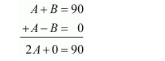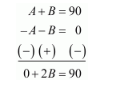# If sin (A + B) = 1 and cos (A − B) = 1,

Question:

If $\sin (A+B)=1$ and $\cos (A-B)=1,0^{\circ} Solution: Given:$\sin (A+B)=1$...(1)$\cos (A-B)=1$....(2) We know that,$\sin 90^{\circ}=1$.....(3)$\cos 0^{\circ}=1$.....(4) Now by comparing equation (1) and (3) We get,$A+B=90 \ldots \ldots(5)$Now by comparing equation (2) and (4) We get,$A-B=0 \ldots \ldots(6)$Now to get the values of A and B, let us solve equation (5) and (6) simultaneously Therefore by adding equation (5) and (6) We get,Therefore,$2 A=90\Rightarrow A=\frac{90}{2}\Rightarrow A=45^{\circ}$Hence$A=45^{\circ}$Now by subtracting equation (6) from equation (5) We get,Therefore,$2 B=90\Rightarrow B=\frac{90}{2}\Rightarrow B=45^{\circ}$Hence$B=45^{\circ}$Therefore the values of A and B are as follows$A=45^{\circ}$and$B=45^{\circ}\$